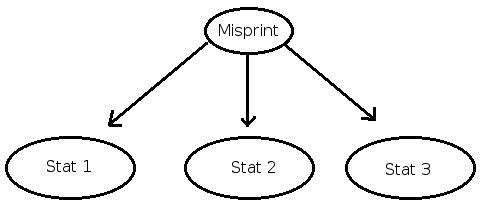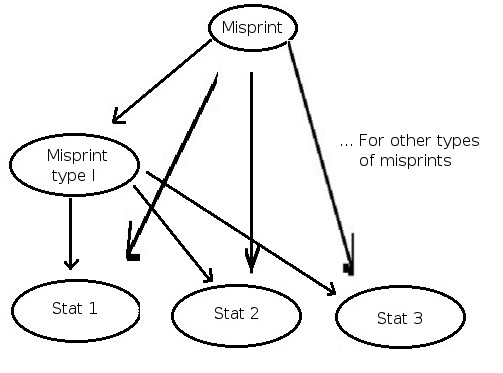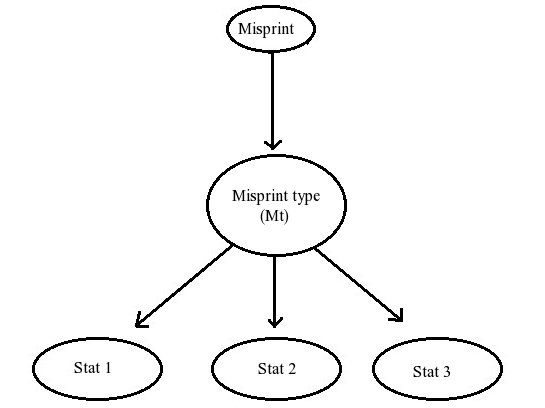Misprint method

(Difference between revisions)
Rjpatruno (Talk | contribs)
(Created page with "On this page you can find details about the algorithm of our misprint detection system. = Statistical methods for misprint detection = With a fast simulator runnin...")

 Statistical methods for misprint detection

With a fast simulator running, we can now develop a general learning algorithm for the misprint detection task, and see how it performs on a simple case. This method becomes practical thanks to the simulator that we build outside ISET, but we can also reuse some of the properties that we have in memory and that we use to simulate the output of the sensor to see if the actual output is reasonable.

As said previously, we have indeed 2 look-up tables:

1. An average image
2. The properties of the deviation from this average.

Our reference image (I_sim: the one that we are simulating), is our best guess of what the sensor output should be, therefore we can directly use the average image. Then we can compare the deviation of the sensed image (I_sensed) from the reference image (I_sim), and compare the measured deviation to the standard deviation of the sensor output for this particular CMYK value. Indeed, we can easily compute, for a random variable, whose probability distribution is known, the cumulative distribution of probability P(x>r*sigma). This gives us an idea of how likely it is for the actual output of the sensor to be r*sigma far from its theoretical average. This give is the following algorithm:

1. Compute I_sim, the average image from the CMYK image (I_in) and with the average look-up table. (==> I_sim)
2. Compute the map of the standard deviations for each pixel (from the other look up table); note: same index in he 2 tables, so this is very fast, no need to research in the lookup table.
3. Sensed the image with the sensor (for us simulate an actual image by adding noise on the average) (==> sensed_image)
4. Compute for a sliding window on the images
• difference(i,j) = |I_sim(i:i+h,j:j+w)-I_sensed(i:i+h,j:j+w)|
• and the theoretical standard deviation of difference: sigma(i,j)
5. Return the map of P(difference(i,j)>sigma(i,j))

This gives us a map of probability (proba_map) over the image and indicates places that are likely and those that are unlikely.

 Statistical learning

We use these statistical maps to extract some image features. From these features we compute the misprint probability. The three statistics (features) that we calculate are: min(proba_map), mean(proba_map) and sum(proba_map<0.5)/size(proba_map) (called fraction).

During a training run, we learn the conditional distribution of the probability of observing each of these features given the binary random variable of a misprint or correct print. Assuming that these features are independent given misprint, we get this simple probability model:Simple statistical model

To learn these conditional probabilities we use an image database to simulate many examples of misprints and correct prints. We compute the features and quantize them to, say, 20 steps. We then count how many times each quantized level occurs given a correct print or misprint. Each quantization level is assigned some positive value (> 0) to avoid problems with zero probability events.

We use this model as follows. We measure new unknown print. We then compute the values of the three features, (min, mean, and fraction). Using Bayes' rule and the data in the table, we calculate P(misprint | min, mean, fraction).

From this model, we can then easily imagine several development to make it more accurate, or to catch several type of misprints A development of this idea that seems quite natural is to add new variables that are the kind of misprint that we have:Developed statistical model

We could also add variables with properties of the image (like the ink repartition, or the presence of some color...)

 A second model

From these comments on the simulation that we did, it seems that a better model could be:The actual modified model that we are using

With the variable Mt = Misprint Type being either:

1. no misprint
2. global misprint
3. local misprint

This should be giving better results as these kind of misprint have a similar effect on the computed value of the features.

We can infer the posterior probability of a misprint given the measured features $P(M|S_{1},S_{2},S_{3})$ with the same kind of calculations than the previous model:

With:

• M=0 <=> correct print
• M=1 <=> misprint
• Mt=0 <=> correct print
• Mt=1 <=> global misprint
• Mt=2 <=> local misprint
• $S = S_{1},S_{2},S_{3}$

$P(M|S) = \sum_{mt=0}^{3}\,P(M|mt,S)\,P(mt|S) = \sum_{mt=0}^{3}\,P(M|mt)\,P(mt|S)$

So

$P(M=1|S) = 0 \cdot P(Mt=0|S)+1 \cdot P(Mt=1|S)+1 \cdot P(Mt=2|S)$

and finally:

$P(M=1|S)\,=\,P(Mt=1|S)+P(Mt=2|S)$

And we know how to compute these 2 terms with the previous method.

We can now see how to more sensors to this method without making new assumptions. This leads to a nice formula that has several good properties:

• We don't have to make more assumptions
• The computation is linear in the number of sensors
• It mostly reuses the one sensor case
• It is completely general in the number of sensors and therefore the code scales very simply

Problem We have a certain CMYK value on the paper: x, a correct value y and n sensors with different QE curves.

The hypothesis (h) that we want to test is: h=0 (misprint) or h=1 (correct print)

for each sensor i, we have a measurement:

$m_{i}(x) = d_{i}(x) + r_{i}(x)$

and we know:

$d_{i}(y)$

Where d is a deterministic function that we know and r is a random variable with 0-mean a variance depending on i and x and from these measurements, we extract for each sensor a set of features:

$s_{i} = G((m_{i}(x)-d_{i}(y))_{(x,y)})$

Where G is a deterministic function of all the measurements and the predictions on the page

What we want to know is:

$P(h|s_{1}, s_{2},...,s_{i},...,s_{n})$

and

$P(h|s_{1}, s_{2},...,s_{i},...,s_{n}) = \frac{P(h,s_{1}, s_{2},...,s_{i},...,s_{n})P(h)}{P(s_{1}, s_{2},...,s_{i},...,s_{n})} = P(s_{1}|h) P(s_{2}|h) ... P(s_{i}|h) ... P(s_{n}|h) \frac{P(h)}{P(s_{1}, s_{2},...,s_{i},...,s_{n})}$

Here, we are using the fact that $P(s_{i}|h)$ are supposed independent, this would be true if we were conditioning on the misprint type because all the noises r are independent and the other functions are deterministic. Here this is not exactly true - as the misprint type can be seen as a hidden random variable that is not accounted for in the model -, but this is the same approximation that we were doing before, considering that the features are independent given misprint or not. It would be truer to condition given the misprint type (considering that no misprint is a misprint type), but this narrows down the scope of the method as it is assuming that we know all the possible misprints.

We can now use the same formula the retrieve the $P(h|s_{i})$ that we already know:

$P(s_{i}|h) = P(h|s_{i}) \frac{P(s_{i})}{P(h)}$

and then:

$P(h|s_{1}, s_{2},...,s_{i},...,s_{n}) = K \frac{P(h|s_{1})P(h|s_{2})...P(h|s_{i})...P(h|s_{n})}{P(h)^{n-1}} = K\,f(h)$

with K a constant in h that we can find with $K(f(0)+f(1)) = 1$ and so finally: $P(h|s_{1}, s_{2},...,s_{i},...,s_{n}) = \frac{f(h)}{f(0)+f(1)}$

As said previously, this requires n computations of P(h|s_{i}) that is our computation in the monochromatic case. It is important to say that without this calculus, we would have to use the cdp table of $P(h|s_{1}, s_{2},...,s_{i},...,s_{n})$, whose size is in $k^{n}$ with k=20 in our case (the number of quantization step for each feature), this clearly becomes untractable.